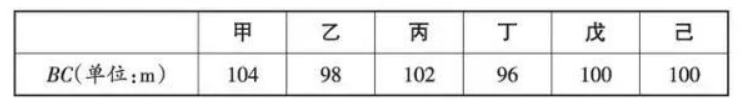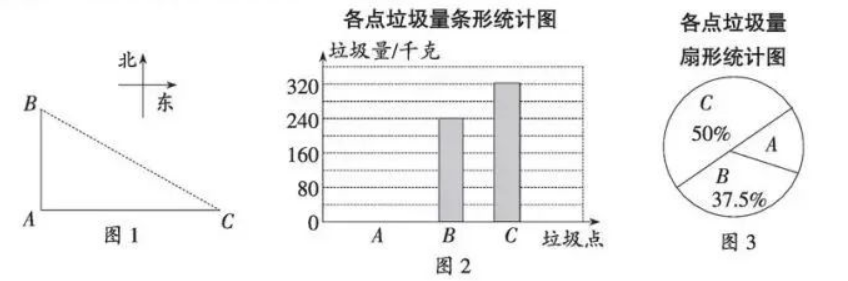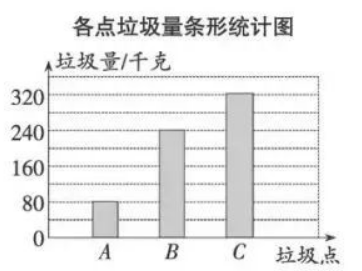(1)表中的中位数是 ,众数是 ,$B C$ 长度的平均数 $\bar{x}=$
(2)求 $A$ 处的垃圾量,并将图 2 补充完整;
(3) 用 (1) 中的 $\bar{x}$ 作为 $B C$ 的长度, 要将 $A$ 处的垃圾沿道路 $A C$ 都运到 $C$ 处, 已知运送 1 千 克垃圾每米的费用为 0.05 元, 求运垃圾所需的费用.(2) $\because C$ 处垃圾量为 $320 \mathrm{~kg}$, 在扇形统计图中所占比例为 $50 \%$,
$\therefore$ 垃圾总量为 $320 \div 50 \%=640(\mathrm{~kg})$,
$\therefore A$ 处垃圾量为 $640-240-320=80(\mathrm{~kg})$,(3) 在直角三角形 $A B C$ 中, $A C=\sqrt{B C^2-A B^2}=\sqrt{100^2-60^2}=80$ (米),
$\because$ 运送 1 千克垃圾每米的费用为 0.05 元,
$\therefore$ 运垃圾所需的费用为 $80 \times 80 \times 0.05=320$ (元).
①点击 收藏 此题， 扫码注册关注公众号接收信息推送（一月四份试卷,中1+高2+研1）
② 程序开发、服务器资源都需要大量的钱，如果你感觉本站好或者受到到帮助，欢迎赞助本站,赞助方式：微信/支付宝转账到 18155261033

 ①此题难易度如何 ②此题推荐度如何 确定